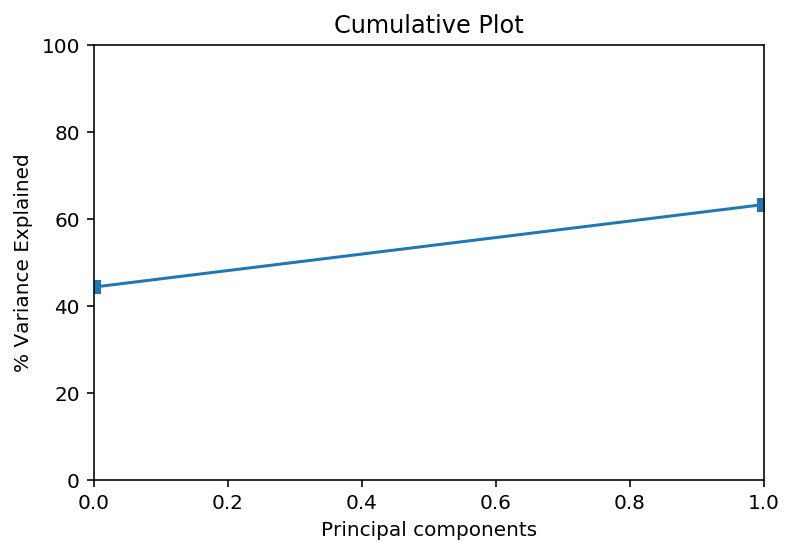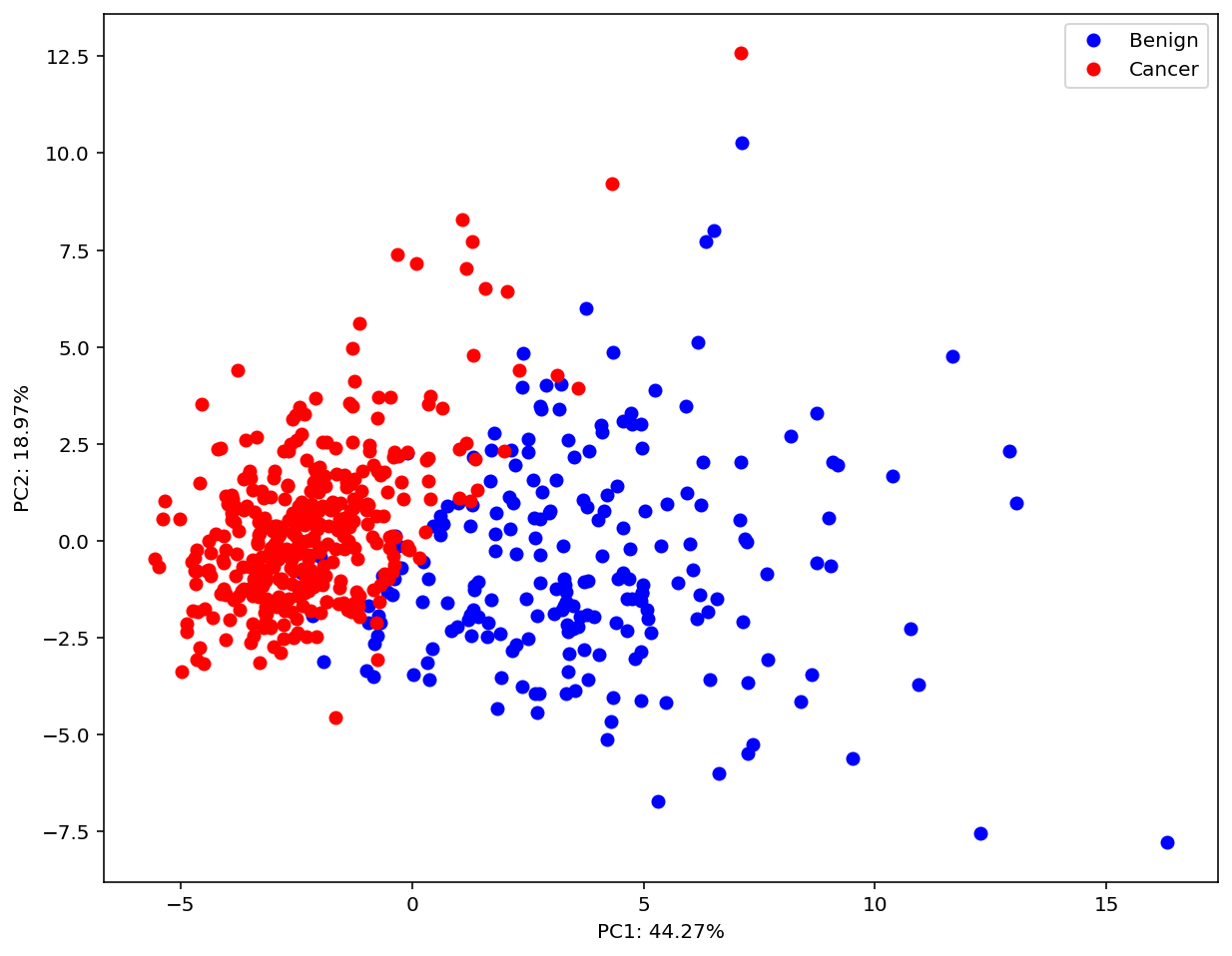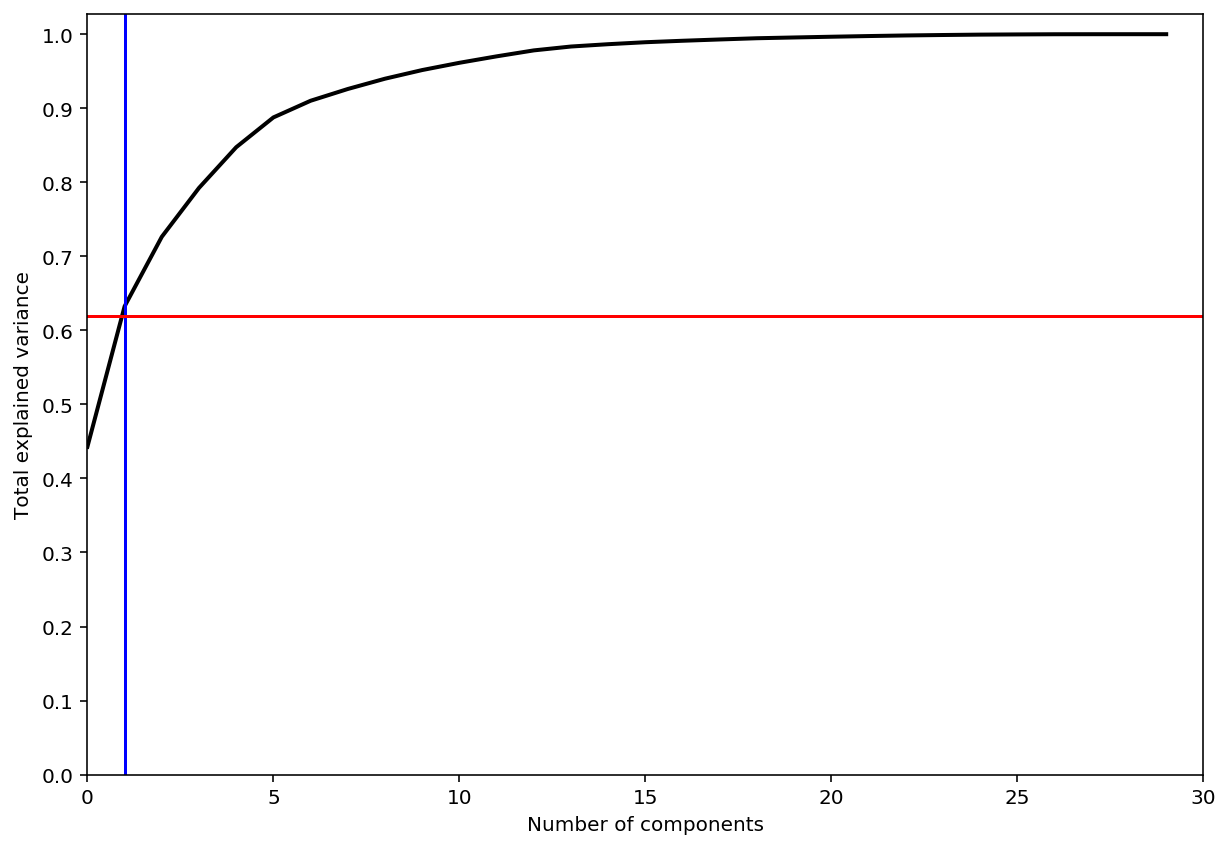# Principal Component Analysis¶

Author: Olatomiwa Bifarin.
PhD Candidate Biochemistry and Molecular Biology
@ The University of Georgia

This is a draft copy, a work in progress

## Notebook Content¶

In :
# More sharp and legible graphics
%config InlineBackend.figure_format = 'retina'


## Background¶

Given that $n$ is the number of samples and $p$ is the number of features; not until recently humans had not have the opportunity to generate dataset where $p>>n$. In other words, we have always had (structured) dataset with many samples but fewer features. High dimensional datasets as they are called - as expected - are immune to traditional statistical treatments, as such galvanizing the innovation of dimensionality reduction techniques. It is one of the popular methods that we will speak to in this short notebook: principal compenent analysis.

In short, it involves projecting $p$ dimensional variables into $M$-dimensional subspace. Just think about it.

All we want to do here is to transform a $p$ dimensional feature space into a lower dimensional space, and guess what? We want to do all these without losing (much) information.

Here is an illustration:Take the illustration above. Notice the almost perfect correlation between $X_{1}$ and $X_{2}$, which suggest informational redundacy. And what will we do if we want to engage in some sort of dimensionality reduction exercise? We project the 2-dimensional feature space (fig A) into one (fig B), and still retain the information we need: variation among the datapoints!

In summary, the aim of PCA is to transform a high dimensional dataset containing potentially correlated features into a small(er) number of uncorrelated features that we will call principal compenents

## Mathematical Description¶

Given a vector $X$:

$$\large X = (x_{1},...x_{p})'$$

We define the variance-covariance matrix of $X$ as the following:

$$\large var(X) = \sum = \begin{bmatrix} \sigma_{1} & \cdots & \cdots & \sigma_{p1}\\ \vdots & \vdots & \ddots & \vdots \\ \sigma_{1p} & \cdots & \cdots & \sigma_{p}^{2}\\ \end{bmatrix} \in \mathbb{R}^{pxp}$$

Now, let's define a series of $Y$ as a linear combinations of $x$ and coefficients $e_{i}$:

$$\large Y_{1} = e_{11}x_{1}+e_{12}x_{2}+...+e_{1p}x_{p}$$$$\large Y_{2} = e_{21}x_{1}+e_{22}x_{2}+...+e_{2p}x_{p}$$$$\large \vdots$$$$\large Y_{p} = e_{p1}x_{1}+e_{p2}x_{2}+...+e_{pp}x_{p}$$

These linear combinations can be thought of as linear regression minus the intercept(bias) term. As such, as stated earlier, $e_{i}$ is the coefficients.

In turn, we can define a covariance matrix of $Y$ as the following:

$$var(Y_{i}) = \textbf e_{i}^{'}\sum \textbf e_{i}^{'}$$

The next mathematical goal is to ask what linear combination of $e_{i}$ maximizes the variance of $Y_{i}.$ The answer to that is that the first principal component is the linear combination that maximizes the variance - accounting for the highest amount of variance as possible in the dataset. The coefficient will be defined as such: $e_{1} = (e_{11},...,e_{1p})'$.

Next, we look for the next set of $e_{2}$ that maximizes the variance of $Y_{2}$ (the maximum amount of variance remaining) with the constraint that $cov(Y_{1},Y_{2})=0$ i.e. $Y_{1}$ and $Y_{2}$ are uncorrelated.

And then the cycles continue.

It seems those coefficients $e_{i}$ holds the key to the solution of our problem. And I do we determine these coefficients? -- eigenvectors.

If $B$ is a square matrix, a non-zero vector $v$ is an eigenvector of $B$ if there is a scalar eigenvalue (λ) such that: $Bv = \lambda v$

It turns out that the solution of the coefficient can be derived via the eigen decomposition of the variance-covariance matrix $\sum$ where $\lambda_{i}$ is the eigen value, and $e_{i}$ is the eigen vector. Such that:

$$\large \sum v = \lambda v$$

with eigen values $$\large \lambda_{1} \geq \lambda_{2}, ..., \lambda_{p}$$ and corresponding eigen vectors. $$\large e_{1}, e_{2}, ..., e_{p}$$

Finally, the variance of $Y_{i}$, that is the $i$-th principal component is equal to the $i$-th $\lambda$:

$$var(Y_{i}) = \lambda_{i} = var(Y_{1} = e_{i1}x_{1}+e_{i2}x_{2}+...+e_{ip}x_{p})$$

## Computations¶

### Dataset¶

In this session, I will be clustering a breast cancer dataset consisting of benign and metastatic data based on the features extracted from the cell nuclei characteristics in the histological images.

In :
# Lets import the  breast cancer wisconsin dataset[link]
import pandas as pd
X = data["data"]
y = data["target"]
feature_names = data["feature_names"]

df = pd.DataFrame(data=X, columns=feature_names)

Out:
mean radius mean texture mean perimeter mean area mean smoothness mean compactness mean concavity mean concave points mean symmetry mean fractal dimension ... worst radius worst texture worst perimeter worst area worst smoothness worst compactness worst concavity worst concave points worst symmetry worst fractal dimension
0 17.99 10.38 122.80 1001.0 0.11840 0.27760 0.3001 0.14710 0.2419 0.07871 ... 25.38 17.33 184.60 2019.0 0.1622 0.6656 0.7119 0.2654 0.4601 0.11890
1 20.57 17.77 132.90 1326.0 0.08474 0.07864 0.0869 0.07017 0.1812 0.05667 ... 24.99 23.41 158.80 1956.0 0.1238 0.1866 0.2416 0.1860 0.2750 0.08902
2 19.69 21.25 130.00 1203.0 0.10960 0.15990 0.1974 0.12790 0.2069 0.05999 ... 23.57 25.53 152.50 1709.0 0.1444 0.4245 0.4504 0.2430 0.3613 0.08758
3 11.42 20.38 77.58 386.1 0.14250 0.28390 0.2414 0.10520 0.2597 0.09744 ... 14.91 26.50 98.87 567.7 0.2098 0.8663 0.6869 0.2575 0.6638 0.17300
4 20.29 14.34 135.10 1297.0 0.10030 0.13280 0.1980 0.10430 0.1809 0.05883 ... 22.54 16.67 152.20 1575.0 0.1374 0.2050 0.4000 0.1625 0.2364 0.07678

5 rows × 30 columns

In :
X = df.values
print('Dimension of the dataset:\n', X.shape)
print('dataset:\n', X)

Dimension of the dataset:
(569, 30)
dataset:
[[1.799e+01 1.038e+01 1.228e+02 ... 2.654e-01 4.601e-01 1.189e-01]
[2.057e+01 1.777e+01 1.329e+02 ... 1.860e-01 2.750e-01 8.902e-02]
[1.969e+01 2.125e+01 1.300e+02 ... 2.430e-01 3.613e-01 8.758e-02]
...
[1.660e+01 2.808e+01 1.083e+02 ... 1.418e-01 2.218e-01 7.820e-02]
[2.060e+01 2.933e+01 1.401e+02 ... 2.650e-01 4.087e-01 1.240e-01]
[7.760e+00 2.454e+01 4.792e+01 ... 0.000e+00 2.871e-01 7.039e-02]]


Standardize datasets

$$z = \large \frac {x-u}{s}$$
In :
from sklearn.preprocessing import StandardScaler
scaler = StandardScaler()
scaler.fit(X)
X_std = scaler.transform(X)

print('Dimension of the dataset:\n', X_std.shape)
print('dataset:\n', X_std)

Dimension of the dataset:
(569, 30)
dataset:
[[ 1.09706398 -2.07333501  1.26993369 ...  2.29607613  2.75062224
1.93701461]
[ 1.82982061 -0.35363241  1.68595471 ...  1.0870843  -0.24388967
0.28118999]
[ 1.57988811  0.45618695  1.56650313 ...  1.95500035  1.152255
0.20139121]
...
[ 0.70228425  2.0455738   0.67267578 ...  0.41406869 -1.10454895
-0.31840916]
[ 1.83834103  2.33645719  1.98252415 ...  2.28998549  1.91908301
2.21963528]
[-1.80840125  1.22179204 -1.81438851 ... -1.74506282 -0.04813821
-0.75120669]]


### p-dimensional mean vector¶

In :
mean_vec = scaler.mean_
print('Dimension of the mean vector:\n', mean_vec.shape)
print('dataset:\n', mean_vec)

Dimension of the mean vector:
(30,)
dataset:
[1.41272917e+01 1.92896485e+01 9.19690334e+01 6.54889104e+02
9.63602812e-02 1.04340984e-01 8.87993158e-02 4.89191459e-02
1.81161863e-01 6.27976098e-02 4.05172056e-01 1.21685343e+00
2.86605923e+00 4.03370791e+01 7.04097891e-03 2.54781388e-02
3.18937163e-02 1.17961371e-02 2.05422988e-02 3.79490387e-03
1.62691898e+01 2.56772232e+01 1.07261213e+02 8.80583128e+02
1.32368594e-01 2.54265044e-01 2.72188483e-01 1.14606223e-01
2.90075571e-01 8.39458172e-02]


### Variance-Covariance Matrix¶

In :
import numpy as np
cov_mat = np.cov(X_std.T)
print('Dimension of variance-covariance matrix:\n', cov_mat.shape)
#print('dataset:\n', cov_mat);

Dimension of variance-covariance matrix:
(30, 30)


### Eigen Decomposition¶

In :
# Eigen Decomposition
e_val, e_vec = np.linalg.eig(cov_mat)

print('Dimension of eigen value vector:', e_val.shape)
print('Dimension of eigen vector matrix:', e_vec.shape)
# print('Eigenvectors \n%s' %eig_vecs)
# print('\nEigenvalues \n%s' %eig_vals)

Dimension of eigen value vector: (30,)
Dimension of eigen vector matrix: (30, 30)


Recall that the eigenvectors with the lowest eigenvalues bear the least information about the data summary; Therefor in order to select the principal components we want, we will rank the eigenvalues from highest to lowest in order choose the top 𝑘 eigenvectors.

In :
e_val = list(e_val)
e_val.sort(reverse=True)

print ('Eigenvalues in descending order:')
for i in e_val:
print(round(i, 2))

Eigenvalues in descending order:
13.3
5.7
2.82
1.98
1.65
1.21
0.68
0.48
0.42
0.35
0.29
0.26
0.24
0.16
0.09
0.08
0.06
0.05
0.05
0.03
0.03
0.03
0.02
0.02
0.02
0.01
0.01
0.0
0.0
0.0


Now let's visualize the percentage of variance by each of the component:

In :
tot = sum(e_val)
var_exp = [(i / tot)*100 for i in sorted(e_val, reverse=True)]
cum_var_exp = np.cumsum(var_exp)

In :
import matplotlib.pyplot as plt

plt.ylabel('% Variance Explained')
plt.xlabel('Principal components')
plt.title('Scree Plot')
#plt.xlim(1,30)
plt.ylim(0,100)
plt.style.context('seaborn-whitegrid')

plt.plot(cum_var_exp, marker='s');


Now, let's take a closer look:

In :
plt.ylabel('% Variance Explained')
plt.xlabel('Principal components')
plt.title('Cumulative Plot')
plt.xlim(0,1)
plt.ylim(0,100)
plt.style.context('seaborn-whitegrid')

plt.plot(cum_var_exp, marker='s');Here, we see that the first 2 components explains ~60% of the variation in the dataset.

Finally, let's use the sklearn to visualize our results.

## Examples¶

In :
from sklearn import decomposition
pca = decomposition.PCA(n_components=2)
X_reduced = pca.fit_transform(X_std)

In :
Var = pca.explained_variance_ratio_ # returns variance ratio of the selected component.
plt.figure(figsize=(10,8))
plt.plot(X_reduced[y == 0, 0], X_reduced[y == 0, 1], 'bo', label='Benign');
plt.plot(X_reduced[y == 1, 0], X_reduced[y == 1, 1], 'ro', label='Cancer');
plt.xlabel("PC1: " + str(round(Var*100, 2)) + "%");
plt.ylabel("PC2: " + str(round(Var*100, 2))+ "%");
plt.legend(loc=0);In :
pca = decomposition.PCA()
X_reduced = pca.fit_transform(X_std)

plt.figure(figsize=(10,7))
plt.plot(np.cumsum(pca.explained_variance_ratio_), color='k', lw=2)
plt.xlabel('Number of components')
plt.ylabel('Total explained variance')
plt.xlim(0, 30)
plt.yticks(np.arange(0, 1.1, 0.1))
plt.axvline(1, c='b')
plt.axhline(0.62, c='r')
plt.show();As shown above ~60% variation in the dataset is captured in this 2D PCA plot.

## Resources and References¶

In [ ]: